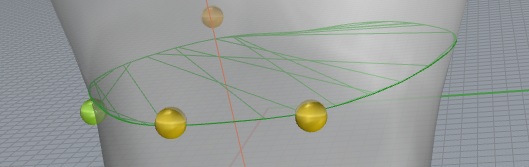# Mesh - Plane intersection contour

Hello,
I want to intersect a cylinder-like mesh (ON_Mesh) with an infinite plane (ON_Plane) and draw the contour (polyline) of the intersection around that mesh.

How can I do that while filtering the points to keep those segments that compose the contour of the intersection? I’ve used ON_Mesh::IntersectPlane() but I get line that connect in the area of the intersection (not just the circumference)

Thanks

Hi @blondbeer,

For contouring specifically, you might consider using MakeRhinoContours.

For example:

cmdSampleSection.cpp

And:

``````CRhinoContourInput input;
input.m_geom.Append(pMesh);
input.m_basept = basept;
input.m_endpt = endpt;
input.m_interval = fabs(interval);
input.m_limit_range = TRUE;

ON_SimpleArray<ON_Polyline*> pline_array;
ON_SimpleArray<ON_Curve*> curve_array;
ON_SimpleArray<ON_3dPoint> point_array;
if (!MakeRhinoContours(input, pline_array, curve_array, point_array))
{
//...
}
``````

Otherwise, we’ll need to see some sample code and a test model.

– Dale

Here’s how I’m computing the intersection:

``````   ON_SimpleArray<ON_Line> ring;
onMesh->IntersectPlane(ringPlane->plane_equation, ring);
``````

and this is how I draw the circumference:

``````      ON_3dPointArray points;
for (auto i = 0; i < ring.Count(); ++i)
{
points.Append(ring[i]);
points.Append(ring[i]);
}
ON_PolylineCurve poly(points);

ON_3dmObjectAttributes attrb;